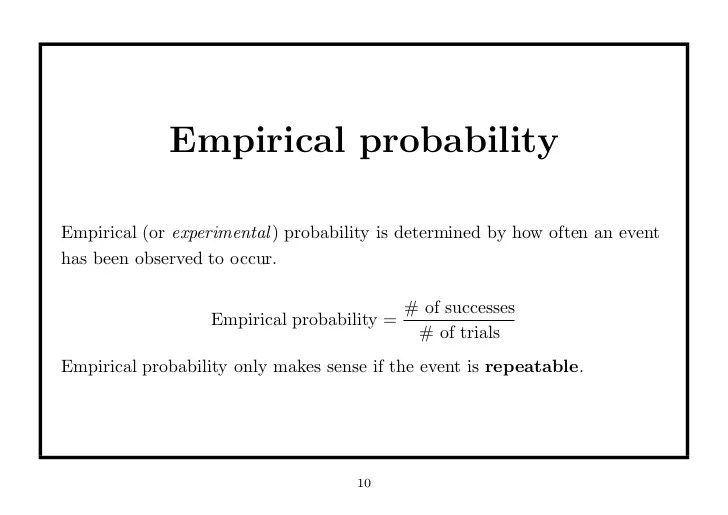# Classical and empirical probability

9th February 2020

Classical Probability: There are ‘n’ number of events and you can find the probability of the happening of an event by applying basic probability formulae. For example – the probability of getting a head in a single toss of a coin is 1/2. This is Classical Probability.Empirical Probability: This type of probability is based on experiments. Say, we want to know that how many times a head will turn up if we toss a coin 1000 times. According to the Traditional approach, the answer should be 500. But according to Empirical approach, we’ll first conduct an experiment in which we’ll toss a coin 1000 times and then we can draw our answer based on the observations of our experiment.